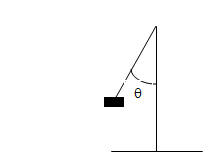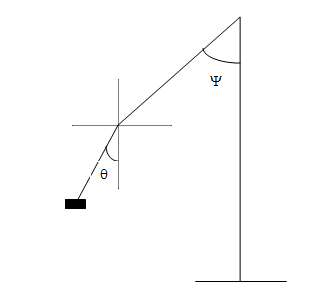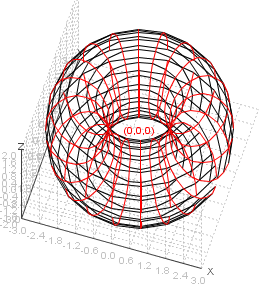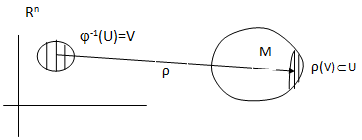# Manifolds

### Basic concepts and principles

Manifold concepts extends to regular surfaces and curves in $\mathbb{R}^n$. Intuitively, a n-dimensional manifold can be considered as a geometric object constructed by "parts of $\mathbb{R}^n$ differentially glued." Where we mean by parts those open sets in the induced usual topology, will be discuss it in detail.

Imagine the simple pendulum.To draw the pendulum position we need a number into $[0, 2 \pi]$ interval: The Circle. $\mapsto$Locally and from topological point of view the circle is like a small interval, a simple piece of line.

Lets increment a dimension, imagine the double pendulum now:To draw the position of double pendulum we need two angles into $[0, 2 \pi] \times [0, 2 \pi]$ intervals: The Torus. $\mapsto$Locally and from topological point of view Torus is a plane (cartesian product of two intervals).

If we still increase one dimension more to consider the triple pendulum we will need tree numbers into $[0, 2 \pi] \times [0, 2 \pi] \times [0, 2 \pi]$ intervals, the object has now 3 dimensions y it is not possible to draw it because it is a a hypersurface we need 4 dimensions: the minimum number to make an (isometric) embedding form this object to into $\mathbb{R}^n$ is n=4).

### Definition of Chart and parameterization

Given a topological space M with the topology T, a chart is a pair $(U, \varphi )$ such us $U \in T$ and $\varphi$ are Homeomorphism

$\varphi : U\subset M \mapsto V\subset \mathbb{R}^n, V=W\cap \varphi(U)$

with W, an open set of $\mathbb{R}^n$. Ie $\varphi(U)$ is open in the topology inherited from the image $\mathbb{R}^n$.
The inverse of the charts $\rho = \varphi^-1$ are called parametrizations.

$\rho : \varphi^{-1}(U) \subset \mathbb{R}^n \mapsto U\subset M$Chart of a Manifold Parametrization of a Manifold

### Maximal (or complete) Atlas Definition

An Maximal (or complete) Atlas $C^\infty$ on M is a chart collection $(U_{\alpha},\varphi _{\alpha})$ such us

1) M⊂ $M \subset \cup_\alpha U_{\alpha}$, ie Uα is a cover of M.

2) Lets $W= \varphi_\alpha(U_{\alpha}) \varphi_\beta(U_{\beta}), W \neq \varnothing$, then, the maps

φαºφβ-1 : φβ(Uβ ) → φα(Uα)

φβºφα-1 : φα(Uα) → φβ(Uβ)

are $C^\infty$.

3) The atlas is maximal,it means that the atlas is not contained in another atlas, ie it contains all the possible charts.
We have all the ingredients for the definition of manifold, simply glue these pieces of Rn.

### Manifold Definition and the Tangent Space

A Manifold C is a Hausdorff topological space dotted with a C maximal atlas .

In a Curve in Rn tangent space is defined as that spanned by the vector tangent to the curve.

In a regular surface Rn tangent space is defined as that generated by two linearly independent tangent vectors of the surface.

In a general manifold there are not environment space, so concept of tangent space is defined in a way a little different. We take as tangent vectors, directional derivative operator in each environment of parametrization, defined by the direction for each coordinate curve, exactly, let x be a point on the manifold M, let $(U, \varphi$ a chart wich coordinate environment containing x and let f be a function of the manifold into Rn, Then we define a tangent vector at x in the direction i as

$\partial_{i}=\frac {\partial(f o \varphi^{-1}) }{\partial x_{i}}$

A tangent vector is then a linear combination of these elements.

So we consider the basis vectors formed by $\{ \partial_{1}, \partial_{2}, ..., \partial_{n} \}$ which generate a vector space at each point p of M, and we call the tangent space of M at p and denote by $T_p(M)$. Therefore

$T_p(M) = SPAN(\{ \partial_{1}, \partial_{2}, ..., \partial_{n} \})$

At the same time, we define the dual of Tp(M), also called cotangent space at p and denoted as
$T_p(M)^*$ which is generated by the basis of covectors.

$T_p(M)^* = SPAN(\{ dx^{1}, dx^{2}, ..., dx^{n} \})$

also called 1-forms.

# Was useful? want add anything?

Post here

### Post from other users

Post here